## Example Questions

### Example Question #601 : Geometry

Quadrilateral ABCD contains four ninety-degree angles. Which of the following must be true?

I. Quadrilateral ABCD is a rectangle.

II. Quadrilateral ABCD is a rhombus.

III. Quadrilateral ABCD is a square.

II and III only

II only

I and II only

I only

I, II, and III

I only

Explanation:

Quadrilateral ABCD has four ninety-degree angles, which means that it has four right angles because every right angle measures ninety degrees. If a quadrilateral has four right angles, then it must be a rectangle by the definition of a rectangle. This means statement I is definitely true.

However, just because ABCD has four right angles doesn't mean that it is a rhombus. In order for a quadrilateral to be considered a rhombus, it must have four congruent sides. It's possible to have a rectangle whose sides are not all congruent. For example, if a rectangle has a width of 4 meters and a length of 8 meters, then not all of the sides of the rectangle would be congruent. In fact, in a rectangle, only opposite sides need be congruent. This means that ABCD is not necessarily a rhombus, and statement II does not have to be true.

A square is defined as a rhombus with four right angles. In a square, all of the sides must be congruent. In other words, a square is both a rectangle and a rhombus. However, we already established that ABCD doesn't have to be a rhombus. This means that ABCD need not be a square, because, as we said previously, not all of its sides must be congruent. Therefore, statement III isn't necessarily true either.

The only statement that has to be true is statement I.

### Example Question #4 : New Sat Math No Calculator

If a polygon has 10 sides, what is the measure of each exterior angle?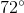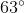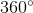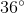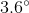Explanation:

In order to figure this out, we need to remember the formula for finding exterior angles.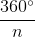, whereis the number of sides of a polygon. Now we simply do the following calculation.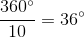So the measure of each exterior angle is.

### All SAT Math Resources